# Data dimensionality reduction

## Wherein I teach myself, amongst other things, feature selection, how a sparse PCA works, and decide where to file multidimensional scaling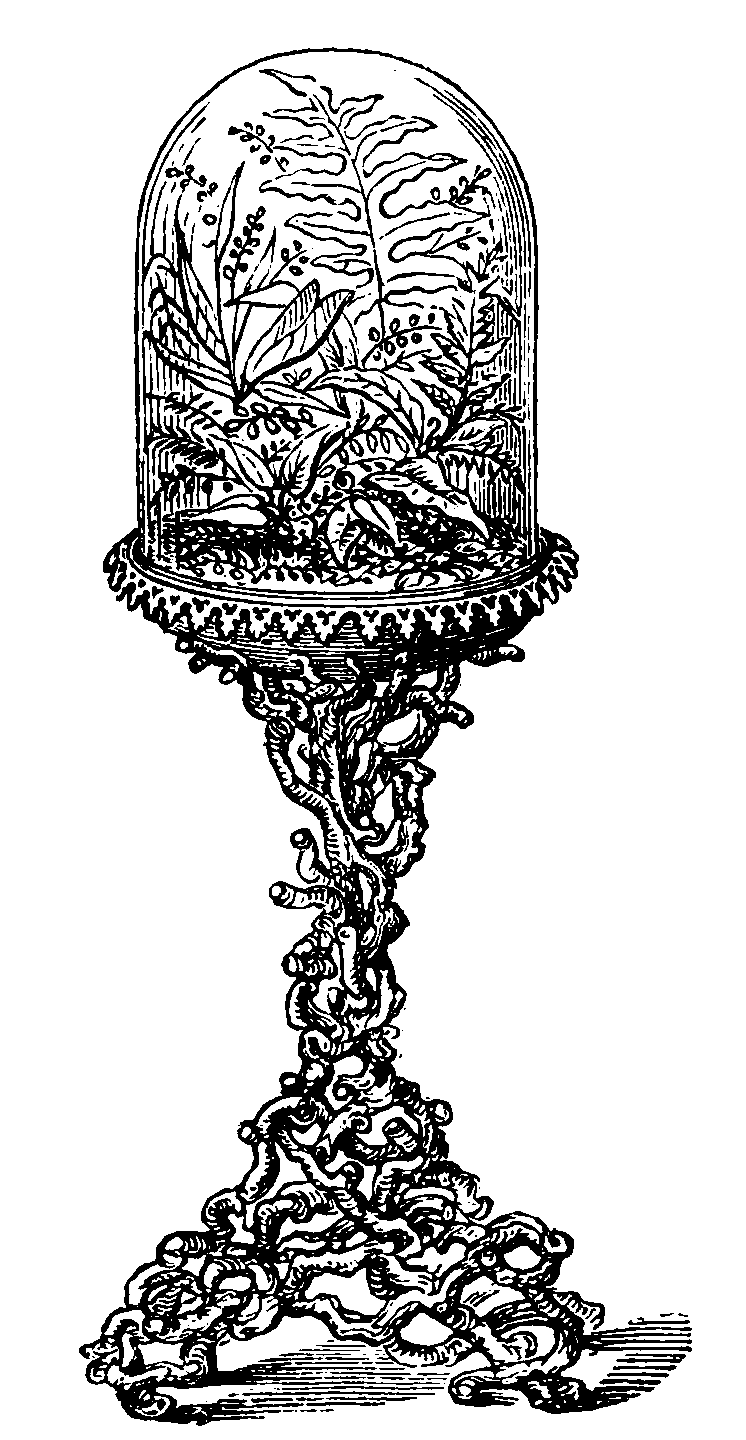🏗🏗🏗🏗🏗

I will restructure learning on manifolds and dimensionality reduction into a more useful distinction.

You have lots of predictors in your regression model! Too many predictors! You wish there were fewer predictors! Maybe then it would be faster, or at least more compact. Can you throw some out, or summarise them in some sens? Also with the notion of similarity as seen in kernel tricks. What you might do to learn an index. Inducing a differential metric. Matrix factorisations and random features, random projections, high-dimensional statistics. Ultimately, this is always (at least implicitly) learning a manifold. A good dimension reduction can produce a nearly sufficient statistic for indirect inference.

## Bayes

Throwing out data in a classical Bayes context is a subtle matter, but it can be done. See Bayesian model selection.

## Learning a summary statistic

See learning summary statistics. As seen in approximate Bayes. Note this is not at all the same thing as discarding predictors; rather it is about learning a useful statistic to make inferences over some more intractable ones.

## Feature selection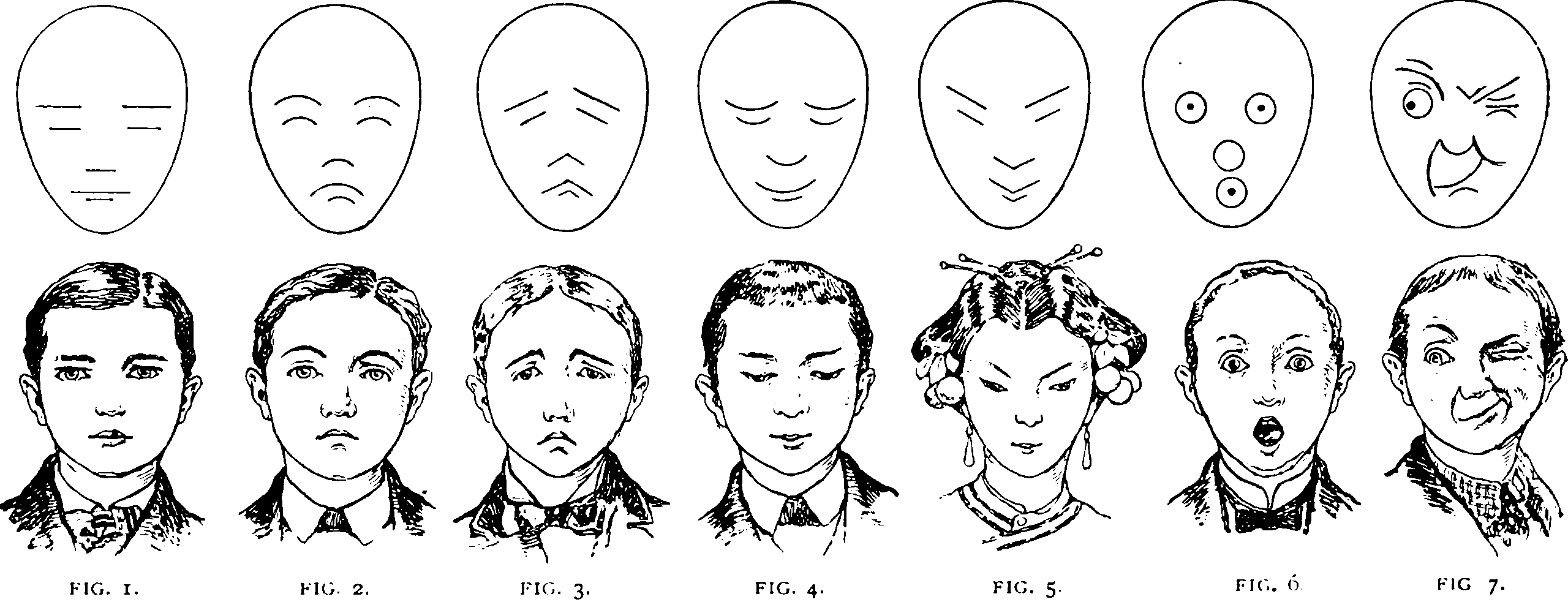Deciding whether to include or discard predictors. This one is old and has been included in regression models for a long time. Model selection is a classic one, and regularised sparse model selection is the surprisingly effective recent evolution. But it continues! FOCI is an application of an interesting new independence test that is very much en vogue despite being in an area that we all thought was thoroughly mined out.

## PCA and cousins

A classic. Has a nice probabilistic interpretation “for free” via the Karhunen-Loève theorem. Matrix factorisations are the family that contains such methods. 🏗

There are various extensions such as additive component analysis:

We propose Additive Component Analysis (ACA), a novel nonlinear extension of PCA. Inspired by multivariate nonparametric regression with additive models, ACA fits a smooth manifold to data by learning an explicit mapping from a low-dimensional latent space to the input space, which trivially enables applications like denoising.

More interesting to me is Exponential Family PCA, which is a generalisation of PCA to non-Gaussian distributions (and I presume to non-additive relations). How does this even work? .

## Learning a distance metric

A related notion is to learn a simpler way of quantifying, in some sense, how similar are two datapoints. This usually involves learning an embedding in some low dimensional ambient space as a by-product.

### UMAP

Uniform Manifold approximation and projection for dimension reduction . Apparently super hot right now. (HT James Nichols). Nikolay Oskolkov’s introduction is neat. John Baez discusses the category theoretic underpinning.

### Locality Preserving projections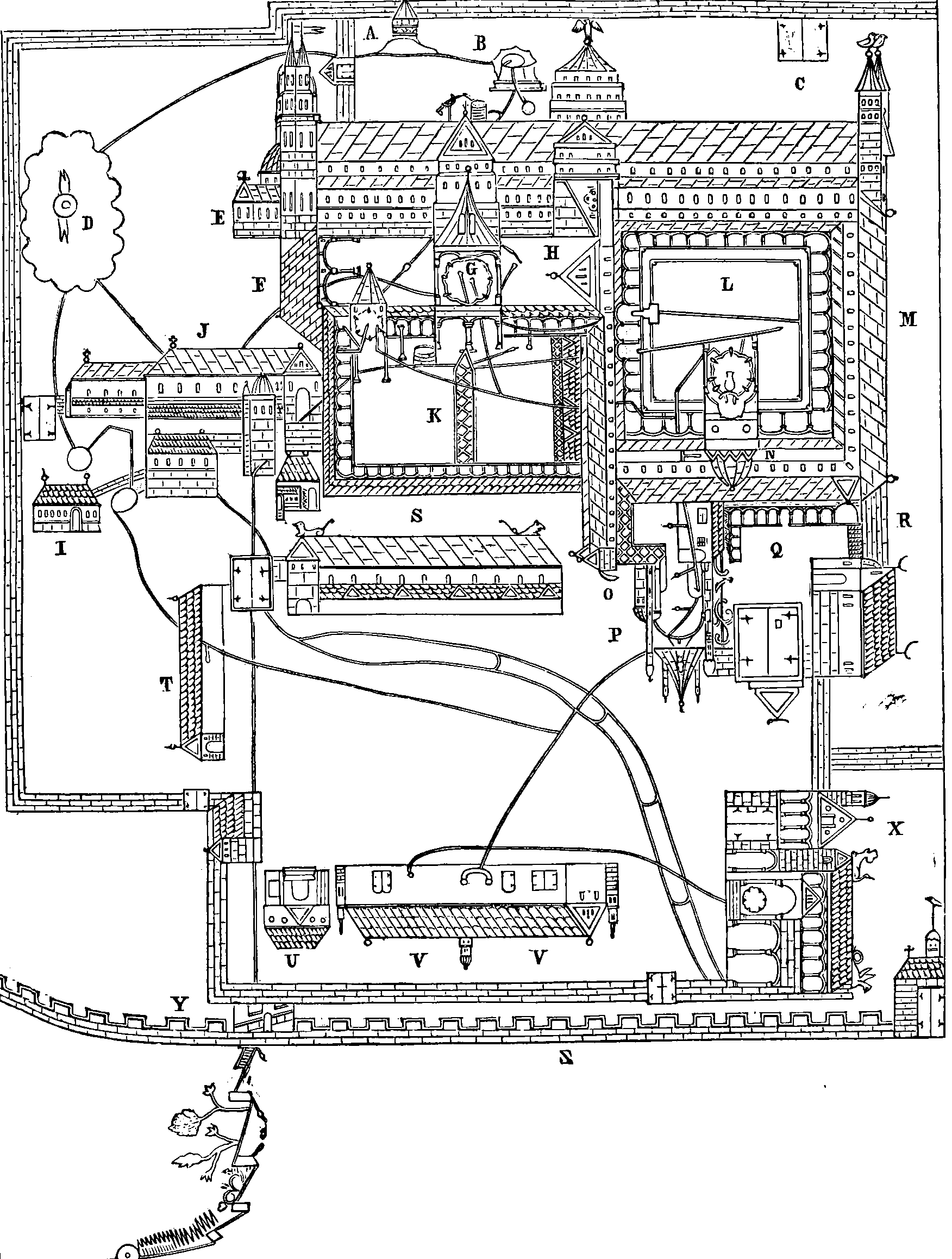Try to preserve the nearness of points if they are connected on some (weight) graph.

$\sum_{i,j}(y_i-y_j)^2 w_{i,j}$

So we seen an optimal projection vector.

(requirement for sparse similarity matrix?)

### Diffusion maps

This manifold-learning technique seemed fashionable for a while.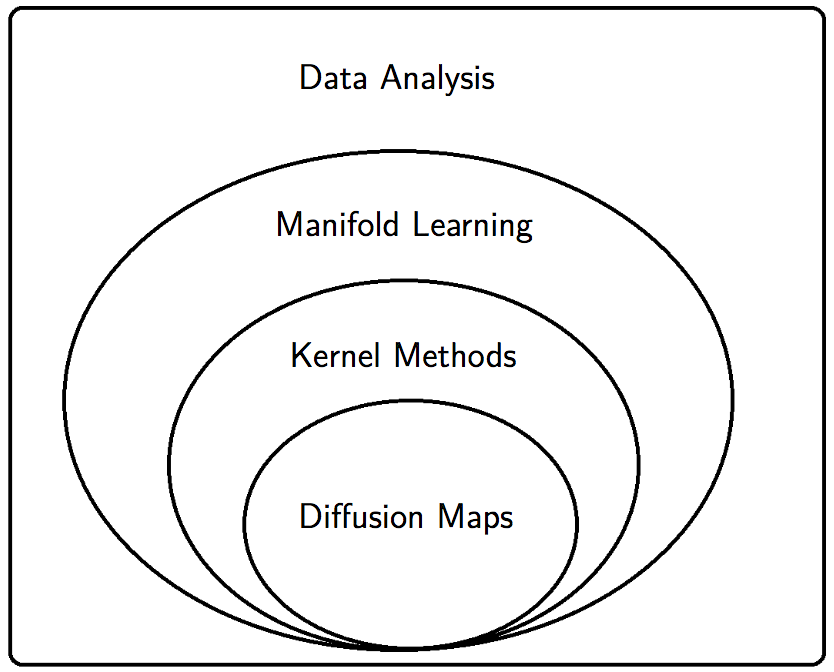Matthew Hirn and Guy Wolf’s taxonomy

Mikhail Belkin connects this to the graph laplacian literature.

### As manifold learning

Same thing, with some different emphases and history, over at manifold learning.

### Multidimensional scaling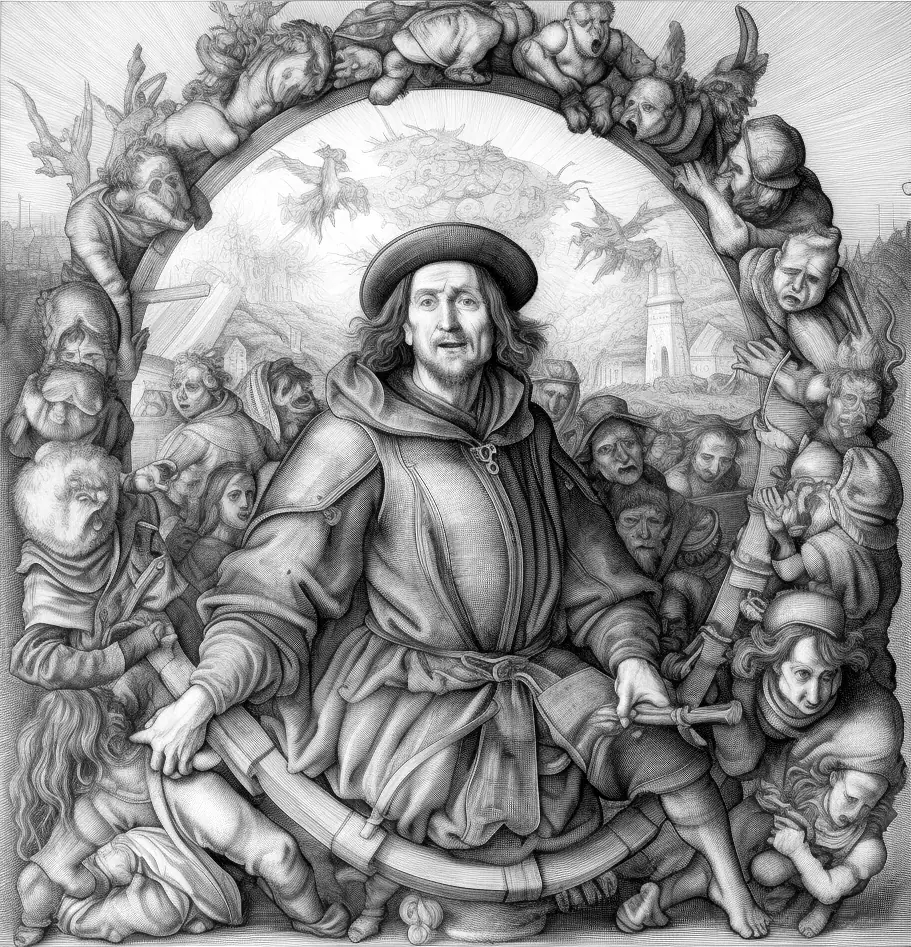TDB.

### Stochastic neighbour embedding and other visualisation-oriented methods

These methods are designed to make high-dimensional data sets look comprehensible in low-dimensional representation.

Probabilistically preserving closeness. The height of this technique is the famous t-SNE, although as far as I understand it has been superseded by UMAP.

Instead of reducing and visualising higher dimensional data with t-SNE or PCA, here are three relatively recent non-linear dimension reduction techniques that are designed for visualising high dimensional data in 2D or 3D:

Trimap and LargeVis are learned mappings that I would expect to be more representative of the original data than what t-SNE provides. UMAP assumes connectedness of the manifold so it’s probably less suitable for data that contains distinct clusters but otherwise still a great option.

## Autoencoder and word2vec

The “nonlinear PCA” interpretation of word2vec, I just heard from Junbin Gao.

$L(x, x') = \|x-x\|^2=\|x-\sigma(U*\sigma*W^Tx+b)) + b')\|^2$

TBC.

## For models rather than data

I do't want to reduce the order of data but rather a model? See model order reduction.

### No comments yet. Why not leave one?

GitHub-flavored Markdown & a sane subset of HTML is supported.